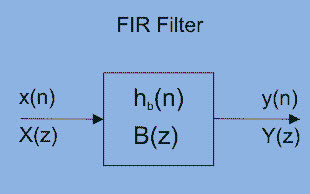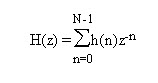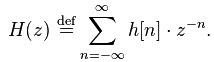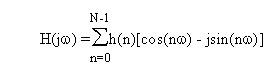# Know all About FIR Filters in Digital Signal Processing

In digital signal processing, an FIR is a filter whose impulse response is of finite period, as a result of it settles to zero in finite time. This is often in distinction to IIR filters, which can have internal feedback and will still respond indefinitely. The impulse response of an Nth order discrete time FIR filter takes precisely N+1 samples before it then settles to zero. FIR filters are most popular kind of filters executed in software and these filters can be continuous time, analog or digital and discrete time. Special types of FIR filters are namely, Boxcar, Hilbert Transformer, Differentiator, Lth-Band and Raised-Cosine.

## What is FIR Filter?The term FIR abbreviation is “Finite Impulse Response” and it is one of two main types of digital filters used in DSP applications. Filters are signal conditioners and function of each filter is, it allows an AC components and blocks DC components. The best example of the filter is a phone line, which acts as a filter. Because, it limits frequencies to a rage significantly smaller than the range of human beings can hear frequencies.

### FIR Filters for Digital Signal Processing

There are various kinds of filters, namely LPF, HPF, BPF, BSF. A LPF allows only low frequency signals through tom its o/p, so this filter is used to eliminate high frequencies. A LPF is convenient for controlling the highest range of frequencies in an audio signal. An HPF is quite opposite to LPF. Because, it rejects only frequency components below some threshold. The best example of the HPF is, cutting out the 60Hz audible AC power, which can be selected up as noise associated almost any signal in the USA.

The alternative of IR filter is a DSP filter which can also be IIR. IIR filters uses feedback, so when you i/p an impulse the o/p theoretically rings forever. The terms used for describing IR filters are Tap, impulse response, MAC (multiply accumulate), delay line, transition band and circular buffer.

### Design Methods of FIR Filter

The design methods of FIR filter based on approximation of ideal filter. The ensuing filter approaches the perfect characteristic because the order of the filter will increase, so creating the filter and its implementation additional complicated.

The design process starts with necessities and specifications the FIR filter. The method used in the design process of the filter depends upon the implementation and specifications. There are many advantages and disadvantages of the design methods. Thus, it is very significant to elect the right method for FIR filter design. Due to efficiency and simplicity of the FIR filter, most commonly window method is used. The other method sampling frequency method is also very simple to use, but there is a small attenuation in the stopband.

#### Logical Structure of FIR Filter

A FIR filter is used to implement almost any type of digital frequency response. Usually these filters are designed with a multiplier, adders and a series of delays to create the output of the filter. The following figure shows the basic FIR filter diagram with N length. The result of delays operates on input samples. The values of hk are the coefficients which are used for multiplication. So that the o/p at a time and that is the summation of all the delayed samples multiplied by the appropriate coefficients.

The filter design can be defined as, it is the process of choosing the length and coefficients of the filter. The intention is to set the parameters so that the required parameters like a stop band and pass band will give the result from running the filter. Most of the engineers use MATLAB software to design the filter.

Usually, filters are defined by their responses to the separate frequency components that found the i/p signal The responses of the filters a classified into three types based on the frequencies such as stop band, pass band and transition band. The response of the passband is the filter’s effect on frequency components that are delivered through mostly unaffected.

Frequencies in a filter’s stopband are, by difference, highly reduced. The transition band signifies the frequencies in the middle, which may receive some reduction, but are not detached completely from the o/p signal.

#### Frequency Response of an FIR Filter

The frequency response plot of the filter is shown below, where ωp is the passband ending frequency, ωs is the stopband beginning frequency, As is the amount of attenuation in the stopband. Frequencies b/n ωp and ωs drop in the transition band and are reduced to some lesser degree.That confirms that the filter meets the preferred specifications includes transition bandwidth, ripple, filter’s length and coefficients. The longer the filter, the more finely the response can be tuned. With the N length and coefficients, float h[N] = {…………}, decided upon, the FIR filter implementation is fairly straightforward.

Z Transform of an FIR Filter is

For an N-tap FIR filter with h(k) coefficient,then the o/p is defined as
y(n)=h(0)x(n) + h(1)x(n-1) + h(2)x(n-2) + ……… h(N-1)x(n-N-1)

The Z-transform of the filter is
H(z)=h(0)z-0 + h(1)z-1 + h(2)z-2 + ……… h(N-1)z-(N-1) orTransfer Function of FIR FilterThe Frequency Response Formula for an FIR FilterDC Gain of an FIR filter isThe applications of FIR filters mainly involve in digital communications in the intermediate frequency stages of the receiver. For instance, a digital radio receives and converts the analog signal to the intermediate frequency and then converts it to digital using with a digital to analog converter. Then uses the finite impulse response to choose the preferred frequency. It is used in software radio, that permits easily adaptable filters with good rejection and without changing hardware.

Thus, this is all about FIR filter, FIR filter design, logical structure and frequency response of FIR filters. We hope that you have got a better understanding of this concept. Furthermore, any queries regarding this topic and applications, please give your suggestions and comments in the comment section below. Here is a question for you, what is the difference between FIR and IIR filter.